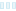# Port C# of RC4 implementation to Java - #CLOSED#

Freelancer: SMikhail
VinderAfvist

Indlæg #1

## Offentlig Præciserings Opslagstavle

•###### SMikhail
• 10 år siden

// Testing code (separate file)

import java.lang.*;
import java.util.*;
import java.io.*;

public class TestRC4
{
public static void main(String[] args)
{
RC4 rc4 = new RC4("mypass", "mytext");
System.out.println(RC4.StrToHexStr(rc4.EnDeCrypt()));

rc4 = new RC4("mypass", RC4.HexStrToStr("AFB9DD0FD3A8"));
System.out.println(rc4.EnDeCrypt());
}
}

• 10 år siden
1.Konkurrenceafholder
• 10 år siden

Hi Mikhail, this looks good. It works with the text values I have I'm just going to get a few more values from a client to test with but it looks fine. The client is in the USA so they won't be awake for about 8 hours so I'll get back to you after that.
I'll post a note on the contest saying I have a working version.
Thanks

J

• 10 år siden
•###### SMikhail
• 10 år siden

// part 3

public static String HexStrToStr(String hexStr)
{
StringBuilder sb = new StringBuilder();
for (int i = 0; i < hexStr.length(); i += 2)
{
int n = Integer.valueOf(hexStr.substring(i, i + 2), 16);
char[] ch = { (char)n };
sb.append(new String(ch));
}
return sb.toString();
}

private void RC4Initialize()
{
sbox = new int[N];
int[] key = new int[N];
for (int a = 0; a < N; a++)
{
sbox[a] = a;
}

int b = 0;
for (int a = 0; a < N; a++)
{
b = (b + sbox[a] + key[a]) % N;
int tempSwap = sbox[a];
sbox[a] = sbox[b];
sbox[b] = tempSwap;
}
}
}

• 10 år siden
•###### SMikhail
• 10 år siden

// part 2
public String EnDeCrypt()
{
RC4Initialize();

int i = 0, j = 0, k = 0;
StringBuilder cipher = new StringBuilder();
for (int a = 0; a < text.length(); a++)
{
i = (i + 1) % N;
j = (j + sbox[i]) % N;
int tempSwap = sbox[i];
sbox[i] = sbox[j];
sbox[j] = tempSwap;

k = sbox[(sbox[i] + sbox[j]) % N];
int cipherBy = (int)(text.charAt(a)) ^ k; //xor operation
char[] ch = { (char)cipherBy };
cipher.append(new String(ch));
}
return cipher.toString();
}

public static String StrToHexStr(String str)
{
StringBuilder sb = new StringBuilder();
for (int i = 0; i < str.length(); i++)
{
int v = (int)str.charAt(i);
String s = Integer.toHexString(v).toUpperCase();
if (s.length() < 2)
sb.append("0");
sb.append(s);
}
return sb.toString();
}

• 10 år siden
•###### SMikhail
• 10 år siden

// part 1

import java.lang.*;
import java.util.*;
import java.io.*;

public class RC4
{
private final static int N = 256;
private int[] sbox;
private String text;

{
this.text = text;
}

{
}

public String getText() { return text; }
public void setText(String value) { text = value; }

•Vis flere kommentarer